Home Practice
For learners and parents For teachers and schools
Textbooks
Full catalogue
Pricing SupportLog in

We think you are located in United States. Is this correct?

# Chapter 13: Physical and chemical change

Matter is all around us. The desks we sit at, the air we breathe and the water we drink are all examples of matter. But matter doesn't always stay the same. It can change in many different ways. In this chapter, we are going to take a closer look at physical and chemical changes that occur in matter.

### Physical changes in matter (ESADT)

A physical change is one where the identities of the substances that are involved do not change. When water is heated for example, the temperature and energy of the water molecules increases and the liquid water evaporates to form water vapour. When this happens, some kind of change has taken place, but the molecular structure of the water has not changed. This is an example of a physical change. All changes in state are physical changes.

$\text{H}_{2}\text{O} \text{(l)} \rightarrow \text{H}_{2}\text{O (g)}$

Conduction (the transfer of energy through a material) is another example of a physical change. As energy is transferred from one material to another, the energy of each material is changed, but not its chemical makeup. Dissolving one substance in another is also a physical change.

Physical change

A change where the chemical identity of a substance does not change. Mass and the number of atoms of each element are both conserved. Physical changes usually involve relatively small changes in energy.

There are some important things to remember about physical changes in matter:

1. Arrangement of particles When a physical change occurs, the compounds may re-arrange themselves, but the bonds in between the atoms will not break. For example when liquid water boils, the molecules will move apart but the molecule will stay intact. In other words water will not break up into hydrogen and oxygen atoms.

Figure 13.1 shows this phase change. Note that the water molecules themselves stay the same, but their arrangement changed.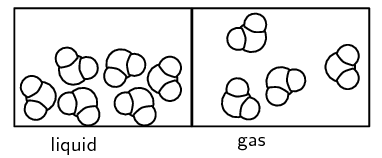Figure 13.1: The arrangement of water molecules in the liquid and gas phase

The bonding of hydrogen and oxygen to form water is explosive and if the water molecule broke apart every time water boiled, life on Earth would not exist for very long!

2. Conservation of mass

In a physical change, the total mass, the number of atoms and the number of molecules will always stay the same. In other words you will always have the same number of molecules or atoms at the end of the change as you had at the beginning.

3. Energy changes

Energy changes may take place when there is a physical change in matter, but these energy changes are normally smaller than the energy changes that take place during a chemical change.

4. Reversibility

Physical changes in matter are usually easier to reverse than chemical changes. Methods such as filtration and distillation can be used to reverse the change. Changing the temperature is another way to reverse a physical change. For example, a mixture of salt dissolved in water can be separated by filtration, ice can be changed to liquid water and back again by changing the temperature.

## Physical change

Use plastic pellets or marbles to represent water in the solid state. What do you need to do to the pellets to represent the change from solid to liquid?

Make a mixture of sand and water. Filter this mixture. What do you observe?

Make a mixture of iron filings and sulfur. Can you separate the mixture with a magnet?

### Chemical changes in matter (ESADU)

When a chemical change takes place, new substances are formed in a chemical reaction. These new products may have very different properties from the substances that were there at the start of the reaction.

Chemical change

The formation of new substances in a chemical reaction. One type of matter is changed into something different.

We will consider some examples of chemical change: the decomposition (breaking down) of hydrogen peroxide and the synthesis (forming) of water and of iron sulfide.

#### Decomposition of hydrogen peroxide

The decomposition (breakdown) of hydrogen peroxide ($$\text{H}_{2}\text{O}_{2}$$) to form water ($$\text{H}_{2}\text{O}$$) and oxygen gas ($$\text{O}_{2}$$) is an example of chemical change. A simplified diagram of this reaction is shown in Figure 13.2. The chemical bonds between $$\text{O}$$ and $$\text{H}$$ in $$\text{H}_{2}\text{O}_{2}$$ are broken and new bonds between $$\text{H}$$ and $$\text{O}$$ (to form $$\text{H}_{2}\text{O}$$) and between $$\text{O}$$ and $$\text{O}$$ (to form $$\text{O}_{2}$$) are formed. A chemical change has taken place.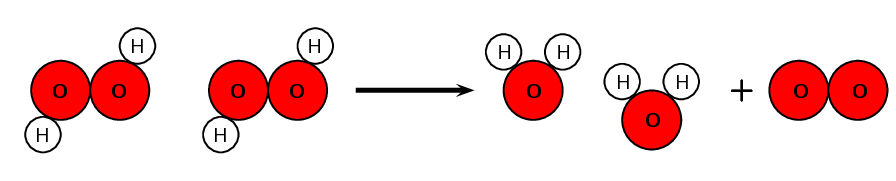Figure 13.2: The decomposition of $$\text{H}_{2}\text{O}_{2}$$ to form $$\text{H}_{2}\text{O}$$ and $$\text{O}_{2}$$

## The decomposition of hydrogen peroxide

### Aim

To observe the decomposition of hydrogen peroxide when it is heated.

### Apparatus

Dilute hydrogen peroxide (about $$\text{3}\%$$); manganese dioxide; test tubes; a flask; a water bowl; stopper and delivery tube, Bunsen burner

Hydrogen peroxide can cause chemical burns. Work carefully with it.

### Method

1. Put a small amount (about $$\text{5}$$ $$\text{mL}$$) of hydrogen peroxide in a flask.

2. Set up the apparatus as shown above.

3. Very carefully add a small amount (about $$\text{0,5}$$ $$\text{g}$$) of manganese dioxide to the flask containing hydrogen peroxide.

### Results

You should observe a gas bubbling up into the second test tube. This reaction happens quite rapidly.

### Conclusions

When hydrogen peroxide is added to manganese dioxide it decomposes to form oxygen and water. The chemical decomposition reaction that takes place can be written as follows:

$2\text{H}_{2}\text{O}_{2}\text{(aq)} \rightarrow 2\text{H}_{2}\text{O (l)} + \text{O}_{2}\text{(g)}$

Note that the manganese dioxide is a catalyst and does not take part in the reaction, so we do not show it in the balanced equation. (A catalyst helps speed up a chemical reaction.)

This experiment used the downward displacement of water to collect a gas. This is a very common way to collect a gas in chemistry. The oxygen that is evolved in this reaction moves along the delivery tube and then collects in the top of the test tube. It does this because it is less dense than water and does not dissolve in water, so the water is displaced downwards.

This reaction is often performed without collecting the oxygen gas and is commonly known as the elephant's toothpaste reaction.

The above experiment can be very vigorous and produce a lot of oxygen very rapidly. For this reason you should use dilute hydrogen peroxide and only a small amount of manganese dioxide.

#### The synthesis of water

The synthesis (forming) of water ($$\text{H}_{2}\text{O}$$) from hydrogen gas ($$\text{H}_{2}$$) and oxygen gas ($$\text{O}_{2}$$) is another example of chemical change. A simplified diagram of this reaction is shown in Figure 13.3. The chemical bonds between $$\text{O}$$ in $$\text{O}_{2}$$ and between $$\text{H}$$ in $$\text{H}_{2}$$ are broken and new bonds between $$\text{H}$$ and $$\text{O}$$ (to form $$\text{H}_{2}\text{O}$$) are formed. A chemical change has taken place.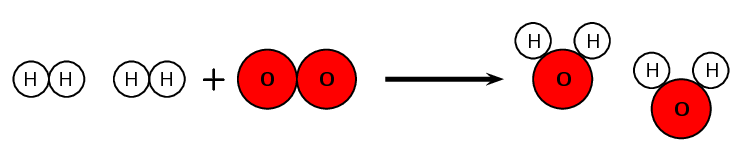Figure 13.3: The synthesis of $$\text{H}_{2}\text{O}$$ from $$\text{H}_{2}$$ and $$\text{O}_{2}$$

## The synthesis of water

### Aim

To observe the synthesis of water.

### Apparatus

Hydrogen gas; balloon; string; candle; long stick: ear plugs; safety glasses

### Method

This reaction can be highly explosive, for this reason it is best done outdoors. Always ensure that you wear ear protection or block your ears. Always have more oxygen than hydrogen in the balloon.

1. Half fill a balloon with hydrogen gas.

2. Fill the remainder of the balloon with oxygen gas.

3. Tie the balloon to one end of the string. Tie down the other end of the string so that the balloon is positioned in mid air, away from any people, objects, walls, ceilings etc.

4. Attach the candle tightly to the stick and light the candle.

5. Standing away from the balloon, carefully hold the candle to the balloon.

### Results

When you bring the candle close to the balloon you should see a flame and hear a loud bang.

### Conclusions

When a mixture of hydrogen and oxygen gas is set alight with a candle a chemical change occurs. Water is made according to the following equation:

$2\text{H}_{2}\text{(g)} + \text{O}_{2}\text{(g)} \rightarrow 2\text{H}_{2}\text{O (l)}$

A mixture of hydrogen and oxygen gas is used as a fuel to get rockets into space.

## The synthesis of iron sulfide

### Aim

To demonstrate the synthesis of iron sulfide from iron and sulfur.

### Apparatus

$$\text{5,6}$$ $$\text{g}$$ iron filings and $$\text{3,2}$$ $$\text{g}$$ powdered sulfur; porcelain dish; test tube; Bunsen burner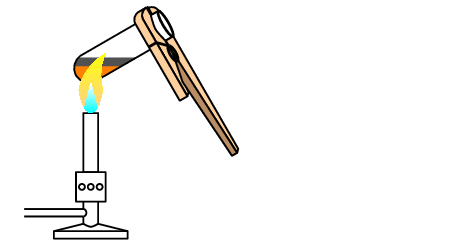### Method

1. Measure the quantity of iron and sulfur that you need and mix them in a porcelain dish.

2. Take some of this mixture and place it in the test tube. The test tube should be about one third full.

3. Heat the test tube containing the mixture over the Bunsen burner. Increase the heat if no reaction takes place. Once the reaction begins, you will need to remove the test tube from the flame. Record your observations.

4. Wait for the product to cool before breaking the test tube with a hammer. Make sure that the test tube is rolled in paper before you do this, otherwise the glass will shatter everywhere and you may be hurt.

5. What does the product look like? Does it look anything like the original reactants? Does it have any of the properties of the reactants (e.g. the magnetism of iron)?

When working with a Bunsen burner, work in a well ventilated space and ensure that there are no flammable substances close by. Always tuck loose clothing in and ensure that long hair is tied back.

### Results

After you removed the test tube from the flame, the mixture glowed a bright red colour. The reaction is exothermic and produces heats. The product, iron sulfide, is a dark colour and does not share any of the properties of the original reactants. It is an entirely new product.

### Conclusions

A synthesis reaction has taken place. The equation for the reaction is:

$\text{Fe (s)} + \text{S (s)} \rightarrow \text{FeS (s)}$

There are some important things to remember about chemical changes:

1. Arrangement of particles

During a chemical change, the particles themselves are changed in some way. In the example of hydrogen peroxide that was used earlier, the $$\text{H}_{2}\text{O}_{2}$$ molecules were split up into their component atoms. The number of particles will change because each $$\text{H}_{2}\text{O}_{2}$$ molecule breaks down into two water molecules ($$\text{H}_{2}\text{O}$$) and one oxygen molecule ($$\text{O}_{2}$$).

2. Energy changes

The energy changes that take place during a chemical reaction are much greater than those that take place during a physical change in matter. During a chemical reaction, energy is used up in order to break bonds and then energy is released when the new product is formed.

3. Reversibility

Chemical changes are far more difficult to reverse than physical changes. When hydrogen peroxide decomposes into water and oxygen, it is almost impossible to get back to hydrogen peroxide.

4. Mass conservation

Mass is conserved during a chemical change, but the number of molecules may change. In the example of the decomposition of hydrogen peroxide, for every two molecules of hydrogen peroxide that decomposes, three molecules are formed (two water and one oxygen).

temp text

Table 13.1 highlights these concepts for the decomposition of hydrogen peroxide.

 $$2\text{H}_{2}\text{O}_{2} \rightarrow 2\text{H}_{2}\text{O} + \text{O}_{2}$$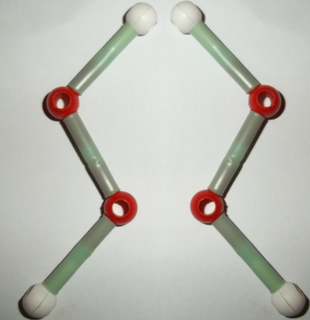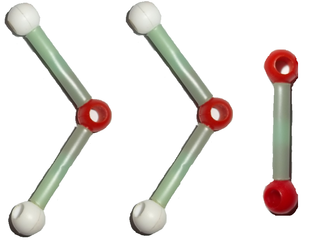Molecules two molecules three molecules Energy changes energy taken in when bonds are broken energy given off when bonds are formed Mass is conserved $$4(\text{1,01}) + 4(\text{16,0}) = \text{68,04}$$ $$2(\text{18,02}) + 2(\text{16,0}) = \text{68,04}$$ Atoms are conserved $$\text{4}$$ oxygen atoms, $$\text{4}$$ hydrogen atoms $$\text{4}$$ oxygen atoms, $$\text{4}$$ hydrogen atoms

Table 13.1: Important concepts in chemical change

## Physical and chemical change

Textbook Exercise 13.1

For each of the following say whether a chemical or a physical change occurs.

1. Melting candle wax.
2. Mixing sodium chloride ($$\text{NaCl}$$) and silver nitrate ($$\text{AgNO}_{3}$$) to form silver chloride ($$\text{AgCl}$$).
3. Mixing hydrochloric acid ($$\text{HCl}$$) and magnesium ribbon ($$\text{Mg}$$) to form magnesium chloride ($$\text{MgCl}_{2}$$).
4. Dissolving salt in water.
5. Tearing a piece of magnesium ribbon.
1. Physical change
2. Chemical change
3. Chemical change
4. Physical change
5. Physical change